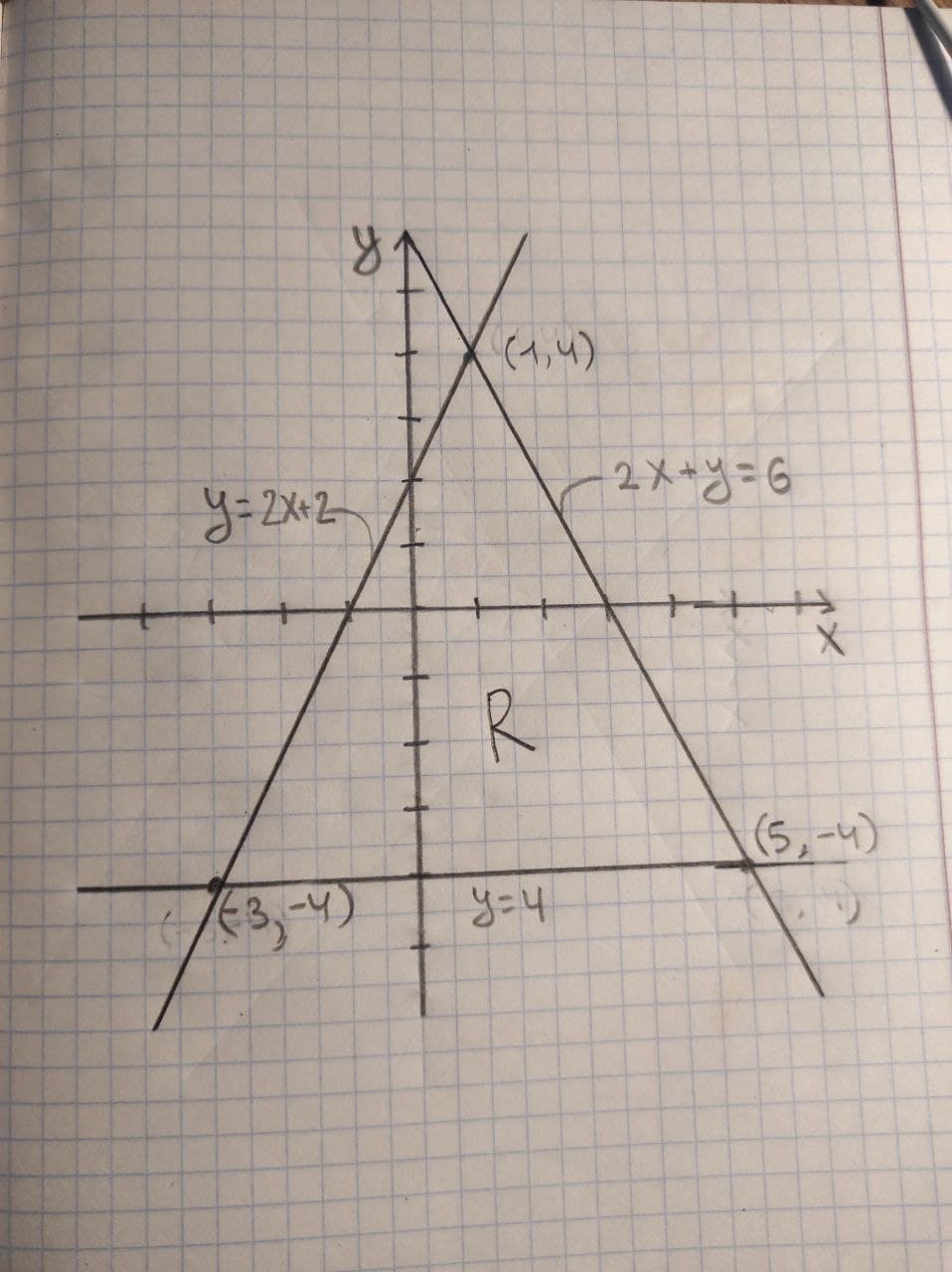# Determine the coordinate of vertices of the figure formed by a given system of inequalities. f(x){(y>=-4),(y<=2x+2),(2x+y<=6):}BolkowN 2021-02-15 Answered
Determine the coordinate of vertices of the figure formed by a given system of inequalities.
$f\left(x\right)\left\{\begin{array}{c}y\ge -4\\ y\le 2x+2\\ 2x+y\le 6\end{array}$
You can still ask an expert for help

## Want to know more about Inequalities systems and graphs?

• Questions are typically answered in as fast as 30 minutes

Solve your problem for the price of one coffee

• Math expert for every subject
• Pay only if we can solve itGennenzip

Draw the line y = -4, now to obtain the region located by $y\ge -4$ take any point and substitute in given inequalities $y\ge -4$ if that point satisfies the inequality, then the region represented by that inequality must contain that point. Take (0,0) and is region above the line y=-4
Draw the line y = 2x+2, now to obtain the region located by $y\le 2x+2$ take any point and substitute in given inequalities $y\le 2x+2$ if that point satisfies the inequality, then the region represented by that inequality must contain that point. Take (0,0) and $0\le 2\cdot 0+2⇒0\le 2$, therefore the region represented by $y\le 2x+2$ is region lies below line y=2x+2 andmust contain point (0,0)
Draw the line 2x+y = 6, now to obtain the region located by 2x+y$\le$ 6 take any point and substitute in given inequalities $2x+y\le 6$ if that point satisfies the inequality, then the region represented by that inequality must contain that point. Take (0,0) and $2\cdot 0+0\le 6⇒0\le 6$, therefore the region represented by $2x+y\le 6$ is region lies below line 2x+y=6 andmust contain point (0,0)
Therefore the graphical representation of given inequalities is shown below: From graph, it is clear that given system of inequalities from a triangle with vertices (1,4), (-3,-4) and (5,-4) R is region enclosed by guve system of inequalities.